# R/qtl2pattern Vignette

#### 2022-01-24

Look for bold stuff to remove or adjust code. Note that changes to summary broke part of qtl2shiny in SNP/Gene Action

This vignette continues an example developed in library qtl2ggplot using data from qtl2data with tools found in qtl2pattern. Calculations are repeated from that example, but only plots that help tell the story. Focus is entirely on chromosome `2` of the DOex from ‘qtl2data’. See vignette in ‘qtl2ggplot’ for complementary analysis.

Package ‘qtl2pattern’ does not depend on ‘qtl2ggplot’, and can be used independently. However this vignette shows some new ‘ggplot2’ based routines that extend ‘qtl2ggplot’ to the functionality of this package. This vignette illustrates these ‘ggplot2’-derived routines, which is why ‘qtl2ggplot’ is suggested.

Package `qtl2pattern` has `summary` methods for `scan1` and `scan1coef`. Not sure these are really needed. The `R/qtl2` routine `find_peaks` takes care of some but not all of this functionality. Sections below are

• Genome Scan Using 36 Allele Pairs
• Genome Scan Using 8 Alleles
• SNP Association Mapping near Peak
• Strain Distribution Pattern (SDP) Scan
``library(qtl2pattern)``

This vignette uses the example Diversity Outbred (DO) data. See Recla et al. (2014). These mice were derived from 8 founder strains, yielding up to 36 allele pairs at any marker. The data can be found in https://github.com/rqtl/qtl2data as `DOex`. While these data span three chromosomes (`2, 3, X`), we focus here on chromosome `2`.

``dirpath <- "https://raw.githubusercontent.com/rqtl/qtl2data/master/DOex"``
``````DOex <-
subset(
chr = "2")``````

``````tmpfile <- tempfile()

Or alternatively calculate those genotype probabilities

``pr <- qtl2::calc_genoprob(DOex, error_prob=0.002)``

## SNP Association Mapping near Peak

This section briefly examines SNP association mapping, which involves collapsing the 36 allele pair genotype probabilities to 3 allele probabilities in the region of a QTL peak.

``````tmpfile <- tempfile()

Rename the SNP position as `pos`, and add SNP index information.

``````snpinfo <- dplyr::rename(snpinfo, pos = pos_Mbp)
snpinfo <- qtl2::index_snps(DOex\$pmap, snpinfo)``````

Convert genotype probabilities to SNP probabilities. Notice that starting with the allele probabilities only ends up with 2 SNP alleles per marker (`"A", "B"`), but we want all three (`"AA" "AB" "BB"`). Recall that the SNP alleles are in context of the strain distribution pattern (`sdp`) for that SNP. The `sdp` for markers (`rsnnn`) are imputed in ‘qtl2’ from the adjacent SNPs. That is, using allele probabilities,

``````apr <- qtl2::genoprob_to_alleleprob(pr)
snpapr <- qtl2::genoprob_to_snpprob(apr, snpinfo)
dim(snpapr[["2"]])``````
``##  261   2 142``
``dimnames(snpapr[["2"]])[]``
``##  "A" "B"``

while using genotype probabilities,

``````snppr <- qtl2::genoprob_to_snpprob(pr, snpinfo)
dim(snppr[["2"]])``````
``##  261   3 142``
``dimnames(snppr[["2"]])[]``
``##  "AA" "AB" "BB"``
``rm(snpapr)``

Perform SNP association analysis (here, ignoring residual kinship).

``scan_snppr <- qtl2::scan1(snppr, DOex\$pheno)``

Package ‘qtl2’ provides a summary of the peak using `qtl2::find_peaks`.

``qtl2::find_peaks(scan_snppr, snpinfo)``
``````##   lodindex       lodcolumn chr      pos      lod
## 1        1 OF_immobile_pct   2 96.88443 7.305389``````

## Strain Distribution Pattern (SDP) Scan

Strain distribution patterns (SDPs) separate out SNPs based on their SDP and plot the top patterns. For instance `sdp = 52` corresponds to pattern `ABDGH:CEF`. That is, the SNP genotype `"AA"` resulting from `qtl2::genoprob_to_snpprob` applied to `pr` corresponds to any of the 36 allele pairs with the two alleles drawn from the reference (`ref`) set of `ABDGH` (15 pairs: `AA, AB, AD, AG, AH, BB, BD, BG, BH, DD, DG, DH, GG, GH, HH`), `"BB"` has two alleles from the alternate (`alt`) set `CEF` (6 pairs: `CC, CE, CF, EE, EF, FF`), and `"AB"` has one from each for the heterogeneous (`het`) set (15 pairs: `AC, AE, ..., HF`). There are 255 possible SDPs, but only a few (4 in our example) that need be examined carefully. One can think of these as a subset of markers for genome scan, where interest is only in those SNPS following a particular `sdp`; as with genome scans, we can fill in for missing data. That is, only a few SNPs may show a particular pattern, but key differences might be seen nearby if we impute SNPs of the same pattern.

The `top_snps_pattern` routine is an extension of `qtl2::top_snps`, which provides more detail on SDPs.

``top_snps_tbl <- top_snps_pattern(scan_snppr, snpinfo)``

This default `summary` is nearly identical to the `summary` of the SNP scan object above:

``(patterns <- summary(top_snps_tbl))``
``````## # A tibble: 4 × 8
##   pheno           min_pos max_pos max_lod min_lod   sdp pattern   snp_id
##   <chr>             <dbl>   <dbl>   <dbl>   <dbl> <int> <chr>     <chr>
## 1 OF_immobile_pct    96.9    97.0    7.31    7.31    52 ABDGH:CEF 3 SNPs
## 2 OF_immobile_pct    96.8    96.8    7.04    7.04    48 ABCDGH:EF 12 SNPs
## 3 OF_immobile_pct    96.9    98.2    5.99    5.99    16 ABCDFGH:E 25 SNPs
## 4 OF_immobile_pct    96.9    96.9    5.97    5.97    20 ABDFGH:CE 9 SNPs``````

There may be multiple SNPs identified for an SDP with the same LOD, covering a range of positions. If there is a range of `lod` values, there are additional SNPs beyond those reported in the summary with `lod` values below the maximum (see `ABDFGH:CE` and `ABCDFGH:E` patterns in plot above). The following summary shows details for the first 10 SNPS with top `lod` per SDP:

``head(summary(top_snps_tbl, "best"))``
``````## # A tibble: 6 × 17
##   pheno    chr     pos   lod snp_id    sdp alleles    AJ    B6 `129`   NOD   NZO
##   <chr>    <chr> <dbl> <dbl> <chr>   <int> <chr>   <dbl> <dbl> <dbl> <dbl> <dbl>
## 1 OF_immo… 2      96.9  7.31 rs5867…    52 C|T         1     1     3     1     3
## 2 OF_immo… 2      96.9  7.31 rs4900…    52 G|A         1     1     3     1     3
## 3 OF_immo… 2      97.0  7.31 rs2445…    52 A|T         1     1     3     1     3
## 4 OF_immo… 2      96.8  7.04 rs2124…    48 C|G         1     1     1     1     3
## 5 OF_immo… 2      96.8  7.04 rs2295…    48 T|A         1     1     1     1     3
## 6 OF_immo… 2      96.8  7.04 rs2543…    48 C|T         1     1     1     1     3
## # … with 5 more variables: CAST <dbl>, PWK <dbl>, WSB <dbl>, interval <int>,
## #   on_map <lgl>``````

## SDP Pattern Scan

A new routine `scan1pattern` scans the peak region for each of the 4 patterns provided. That is, the SDP scan only considers SNPs that have a particular strain distribution pattern and uses the SNP probabilities. However, for each markers (or any genome position), one can impute a SNP with any particular SDP and compute its SDP probabilities. That is, we could consider the SDP probabilities at every marker position for the SDP 48 (pattern `ABCDGH:EF`) and do an SDP scan with these SDP probabilities. That is what the routine `scan1pattern` does.

``````scan_pat <- scan1pattern(pr, DOex\$pheno,
map = DOex\$pmap,
patterns = patterns)``````
``summary(scan_pat, DOex\$pmap)``
``````## # A tibble: 4 × 7
##     sdp snp_id  max_pos pheno           founders  contrast max_lod
##   <int> <chr>     <dbl> <chr>           <chr>     <chr>      <dbl>
## 1    52 3 SNPs     98.3 OF_immobile_pct ABDGH:CEF ""          7.31
## 2    48 12 SNPs    98.3 OF_immobile_pct ABCDGH:EF ""          7.04
## 3    16 25 SNPs    98.3 OF_immobile_pct ABCDFGH:E ""          5.99
## 4    20 9 SNPs     96.8 OF_immobile_pct ABDFGH:CE ""          5.97``````

Here is a scan around the region where we have SNP information. The SDPs 52 and 53 are both higher than the other two patterns, and sustain the SDP peak several cM to the right of the allele peak region. This difference in pattern, if real, might suggest some form of allele interaction is important.

``pat_names <- paste(c(52,43,16,20), colnames(scan_pat\$scan), sep = "_")``
``````plot(scan_pat\$scan, DOex\$pmap, lodcolumn = 2, col = "red", xlim = c(90,110), type = "b")
abline(v = c(96.5, 98.5), col = "darkgray", lwd = 2, lty = 2)
plot(scan_pat\$scan, DOex\$pmap, lodcolumn = 1, add = TRUE, col = "blue", type = "b")
plot(scan_pat\$scan, DOex\$pmap, lodcolumn = 3, add = TRUE, col = "purple", type = "b")
plot(scan_pat\$scan, DOex\$pmap, lodcolumn = 4, add = TRUE, col = "green", type = "b")
title("Scans for SDP 52 (blue), 43 (red), 16 (purple), 20 (green)")``````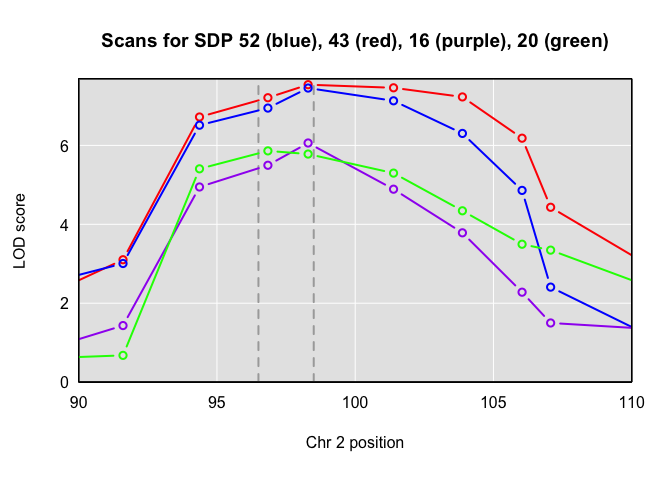Here is a scan over the whole chromosome, imputing SDP. These correspond to reducing the 8 alleles to 2 corresponding to the `ref` (`ABDGH` for sdp 52) and `alt` (`CEF` for sdp 52) composite alleles for the blue curve.

``````plot(scan_pat\$scan, DOex\$pmap, lodcolumn = 2, col = "red")
abline(v = c(96.5, 98.5), col = "darkgray", lwd = 2, lty = 2)
plot(scan_pat\$scan, DOex\$pmap, lodcolumn = 1, add = TRUE, col = "blue")
plot(scan_pat\$scan, DOex\$pmap, lodcolumn = 3, add = TRUE, col = "purple")
plot(scan_pat\$scan, DOex\$pmap, lodcolumn = 4, add = TRUE, col = "green")
title("Scans for SDP 52 (blue), 43 (red), 16 (purple), 20 (green)")``````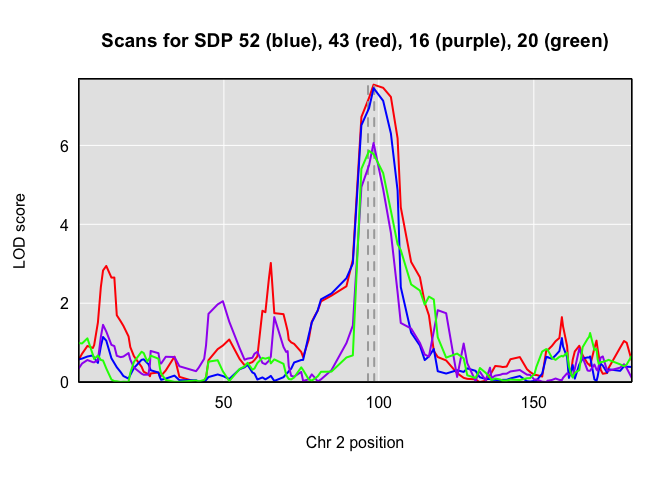### SDP Effects Scan

In a similar fashion to a two-allele cross, we can examine the SDP effects. The top row of the plot below is for the patterns with the higher LOD score.

``````oldpar <- par(mfrow = c(2,2))
cols <- c("blue","purple","red")
for(i in 1:4) {
plot(scan_pat\$coef[[i]], DOex\$pmap, columns = 1:3, col = cols, xlim = c(90,110), type = "b")
title(paste("Coefficients for", pat_names[i]))
if(i == 3) {
abline(v = c(96.5, 98.5), col = "darkgray", lwd = 2, lty = 2)
legend(104, -3, legend = c("ref","het","alt"),
col = cols, lty = 1, lwd = 2)
}
}``````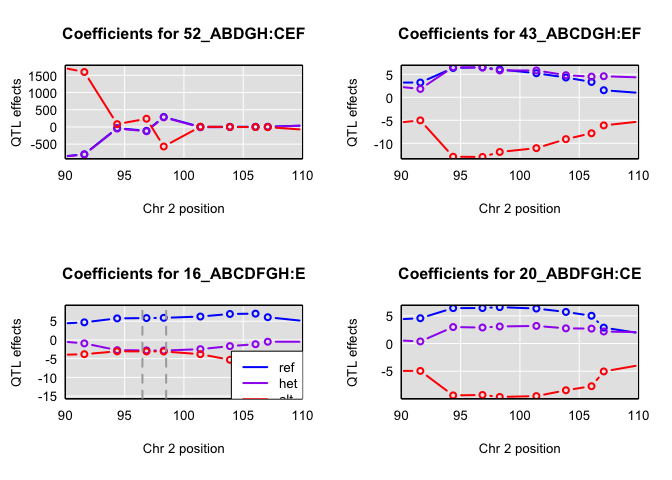``par(oldpar)``

From these, the coefficients for pattern `ABDGH:CEF` (sdp 52) appear to be problematic, whereas pattern `ABCDGH:EF` show a clear pattern of dominance of the reference allele, consistent with the earlier plot of allele coefficients where `E = NZO` and `F = CAST`. The pattern `ABCFGH:CE` shows a similar dominance, but the allele `C = 129` does not stand out in the allele plot. This could be that `129` is important in combination with `NZO`. This is even more apparent when looking at coefficients over the entire chromosome.

``````par(mfrow = c(2,2))
cols <- c("blue","purple","red")
for(i in 1:4) {
plot(scan_pat\$coef[[i]], DOex\$pmap, columns = 1:3, col = cols)
title(paste("Coefficients for", pat_names[i]))
if(i == 3) {
abline(v = c(96.5, 98.5), col = "darkgray", lwd = 2, lty = 2)
legend(125, -3, legend = c("ref","het","alt"),
col = cols, lty = 1, lwd = 2)
}
}``````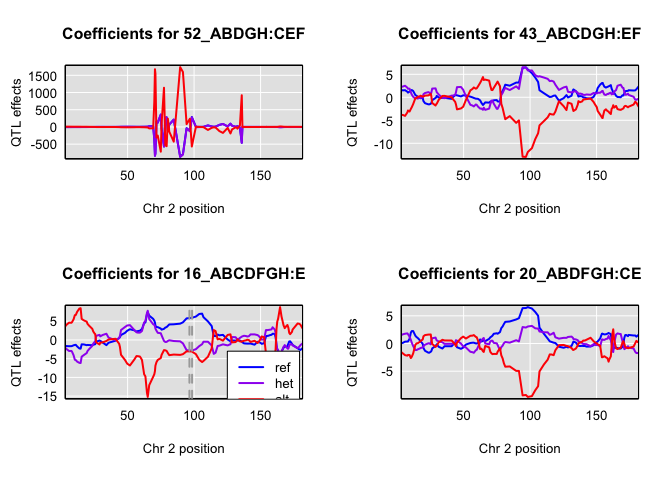### 36 Allele Pair Scan

We are now going to look at some of the 36 coefficients. These are unwieldy, so we collapse the allele pairs for SDP 52, pattern `ABCDGH:EF` into `"ref", "het", "alt"` for summaries, looking individually only at pairs `"EE", "EF", "FF"`.

``coefs2 <- qtl2::scan1coef(pr, DOex\$pheno[,"OF_immobile_pct"], zerosum = FALSE)``

The following is messy code to just pull out weighted averages corresponding to reference, het, and alternative genotypes at the `pr` peak.

``````x <- LETTERS[1:8]
ref <- outer(x,x,paste0)
ref <- ref[upper.tri(ref, diag=TRUE)]
ss <- 2 - sapply(stringr::str_split(ref, ""), function(x) x == x)
alt <- ref[!stringr::str_detect(ref, c("A|B|C|D|G|H"))]
het <- ref[stringr::str_detect(ref, c("A|B|C|D|G|H")) & stringr::str_detect(ref, c("E|F"))]
altw <- ss[match(alt, ref, nomatch = 0)]
hetw <- ss[match(het, ref, nomatch = 0)]
refw <- ss[!stringr::str_detect(ref, c("E|F"))]
ref <- ref[!stringr::str_detect(ref, c("E|F"))]``````
``````coefsum <- coefs2
coefsum[,"AA"] <- apply(coefsum[,ref], 1, weighted.mean, w = refw)
coefsum[,"AB"] <- apply(coefsum[,het], 1, weighted.mean, w = hetw)
coefsum[,"BB"] <- apply(coefsum[,alt], 1, weighted.mean, w = altw)
colnames(coefsum)[1:3] <- c("ref","het","alt")``````
``````plot(coefsum, DOex\$pmap, c("ref", "het", "alt", alt), xlim = c(90,110), ylim = c(-500, 500),
col = c(1,8,7,2:4), type = "b")
abline(h = mean(DOex\$pheno[,"OF_immobile_pct"]), lwd = 2, col = "darkgrey", lty = 2)
abline(v = c(96.5, 98.5), col = "darkgray", lwd = 2, lty = 2)
title("Allele Coefficients for OF_immobile_pct")
legend(105, 80, legend = c("ref", "het", "alt", alt),
col = c(1,8,7,2:4), lty = 1, lwd = 2)``````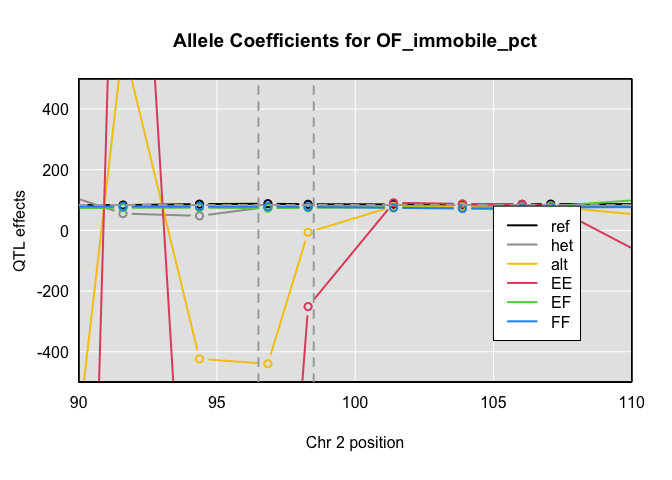Estimates for allele pairs are imprecise, so let’s drop the extreme `"EE"`. The `"ref"`, `"het"` and `"alt"` are weighted mean of 15, 6, 15 allele pairs, respectively, assuming Hardy-Weinberg equilibrium. We see that the allele pairs `"EF", "FF"` lie near `"het"` and below `"ref"` in the peak region in the graph below.

``````plot(coefsum, DOex\$pmap, c("ref", "het", alt[-1]), xlim = c(90,110), ylim = c(55,90),
col = c(1,8,3:4), type = "b")
abline(h = mean(DOex\$pheno[,"OF_immobile_pct"]), lwd = 2, col = "darkgrey", lty = 2)
abline(v = c(96.5, 98.5), col = "darkgray", lwd = 2, lty = 2)
title("Allele Pair Coefficients for OF_immobile_pct")
legend(107, 75, legend = c("ref", "het", alt[-1]),
col = c(1,8,3:4), lty = 1, lwd = 2)``````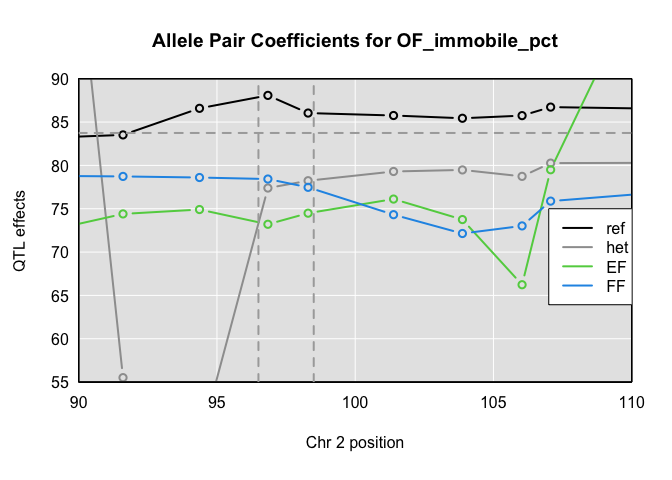### Effect estimates

These look off somehow. Probably don’t have best choice of position. Also, allele1 is fragile if you don’t provide stuff. Also, want to add plot of data.

``````scan_apr <- qtl2::scan1(apr, DOex\$pheno)
coefs <- qtl2::scan1coef(apr, DOex\$pheno)
coefs2 <- qtl2::scan1coef(pr, DOex\$pheno)``````
``````alleles <- allele1(probD = pr,
scanH = scan_apr, coefH = coefs, coefD = coefs2,
scan_pat = scan_pat, map = DOex\$pmap, alt = "E")``````
``ggplot2::autoplot(alleles, scan_apr, DOex\$pmap, frame = FALSE)``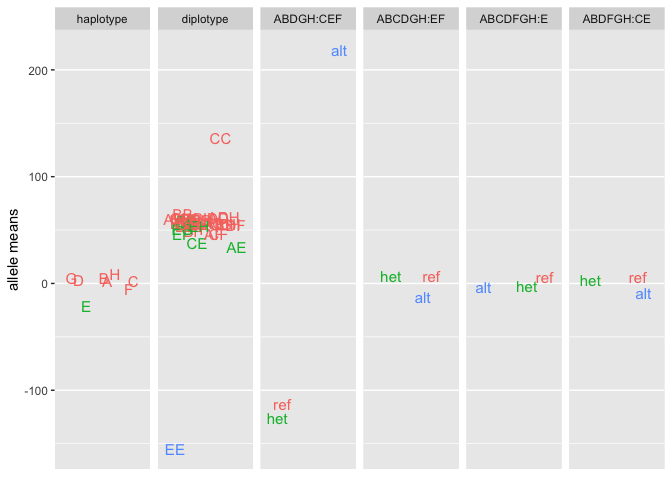Look at subset with haplotype and the 3 well-behaved SDPs.

``````aa <- subset(alleles, sources = levels(alleles\$source)[c(1,4:6)])
ggplot2::autoplot(aa, scan_apr, DOex\$pmap, frame = FALSE)``````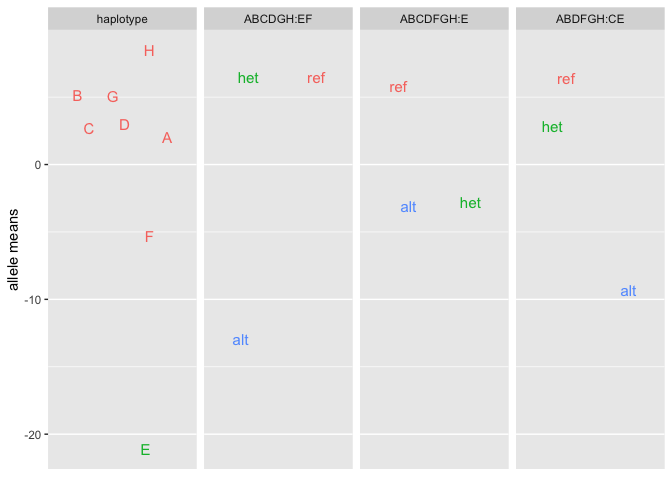The following plot of data uses the closest marker to the SDP 48 max. Hopefully this marker has SDP 48. We could likely improve things with more work by using the SDP probabilities. Check sign on calculations.

``````pos = patterns\$max_pos[patterns\$sdp == 48]
wh <- which.min(abs(pos - DOex\$pmap[]))
geno <- apply(snppr[][,,wh], 1, function(x) which.max(x))
geno <- factor(c("ref","het","alt")[geno], c("ref","het","alt"))
dat <- data.frame(DOex\$pheno, geno = geno)
dat\$x <- jitter(rep(1, nrow(dat)))``````
``````ggplot2::ggplot(dat) +
ggplot2::aes(x = x, y = OF_immobile_pct, col = geno, label = geno) +
ggplot2::geom_boxplot() +
ggplot2::geom_point() +
ggplot2::facet_wrap(~ geno)``````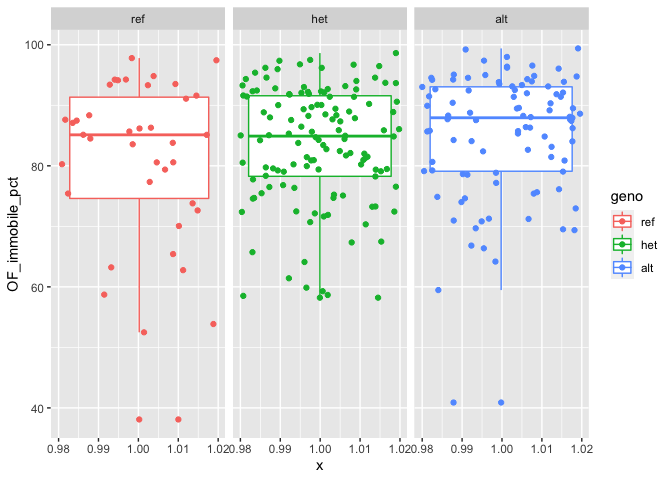These plots anticipate some of the possibilities with mediation.

## SNP features and Gene action

Download Gene info for DOex from web via RDS. For the example below, genes are presented without exons.

``````tmpfile <- tempfile()
class(gene_tbl) <- c("feature_tbl", class(gene_tbl))``````

Variants are usually SNPs, but may be of other types, such as deletions (`DEL`), insertions (`INS`), or insertions and deletions (`InDel`). The routine cannot yet handle major chromosomal rearrangements and translocations. In addition variants may be intronic, exonic, or intergenic, depending on the “consequence” as reported in the variant database.

``````out <- merge_feature(top_snps_tbl, snpinfo, scan_snppr, exons = gene_tbl)
summary(out, "pattern")``````
``````##      ABCDFGH:E ABCDGH:EF ABDFGH:CE ABDGH:CEF
## [1,]        25        12         9         3``````

``out\$snp_type <- sample(c(rep("intron", 40), rep("exon", nrow(out) - 40)))``
``ggplot2::autoplot(out, "OF_immobile_pct")``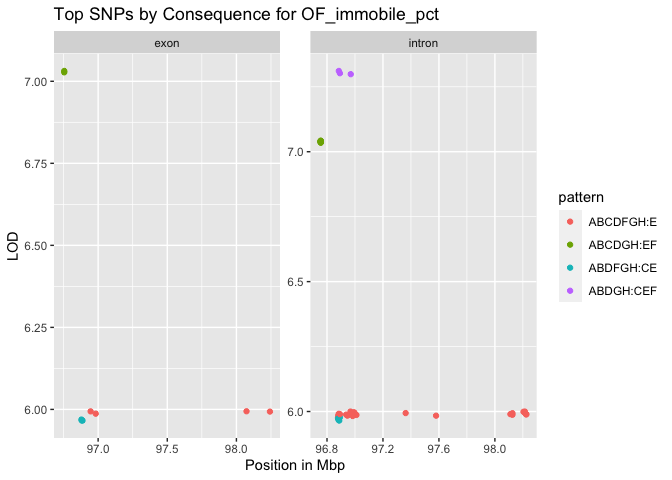``ggplot2::autoplot(out, "OF_immobile_pct", "consequence")``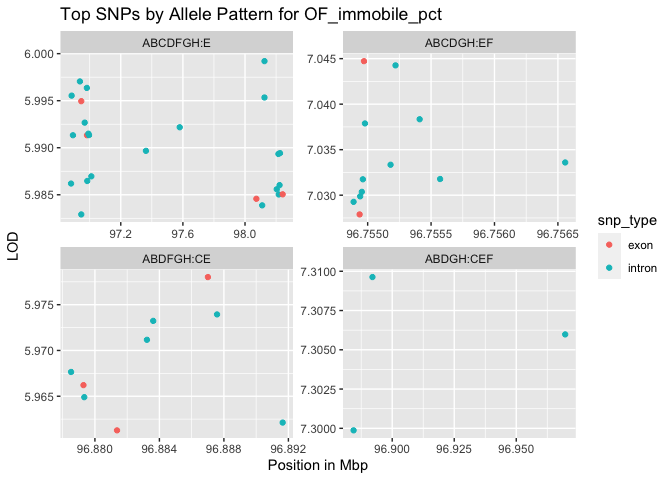It is also possible to consider different types of gene action for SDPs. With no restrictions, there are 2 degrees of freedom (`add+dom`). Various one df options include `additive`, `dominant`, `recessive` and `non-add`itive. It is also possible if sex is encoded to separate analysis by sex.

This currently ignores snp_type

## Gene and exon scan

``````# Not quite right.
gene <- "Lrrc4c"
geneinfo <- dplyr::filter(gene_tbl, Name == gene)
parts <- geneinfo\$start + diff(unlist(geneinfo[,c("start","stop")])) * (0:5) / 5
geneinfo <- geneinfo[rep(1,3),]
geneinfo\$type <- "exon"
geneinfo\$start <- parts[c(1,3,5)]
geneinfo\$stop <- parts[c(2,4,6)]
gene_tbl <- rbind(gene_tbl[1,], geneinfo, gene_tbl[-1,])``````

Plot Genes within some distance of high SNPs.

``qtl2::plot_genes(gene_tbl, xlim = c(96,99))``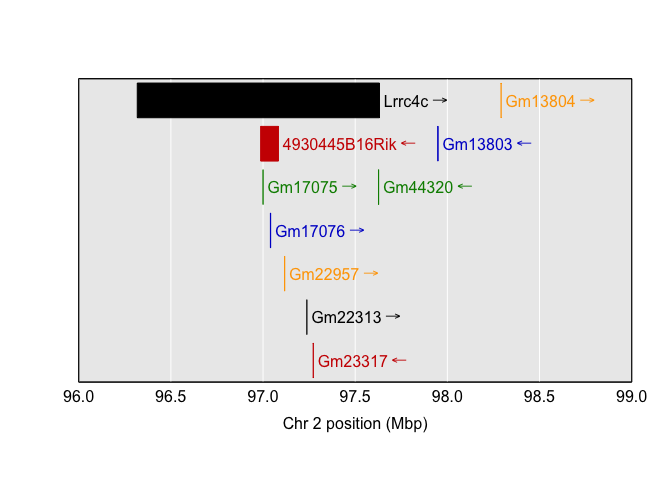``ggplot2::autoplot(gene_tbl)``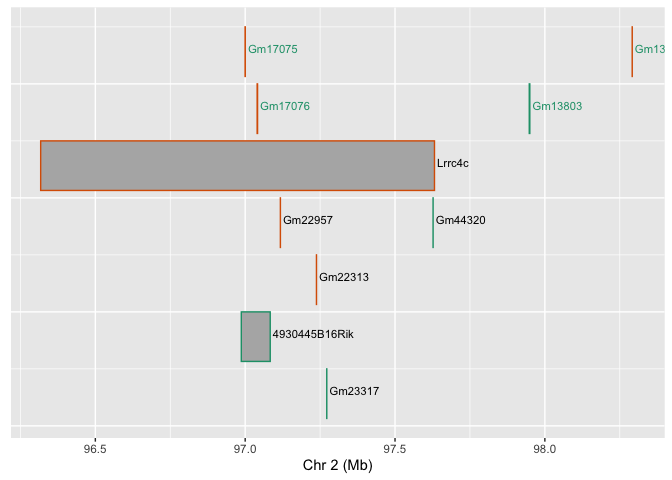Adding the result of `top_snps_pattern` overlays significant SNPS on the plot of genes.

``ggplot2::autoplot(gene_tbl, top_snps_tbl = top_snps_tbl)``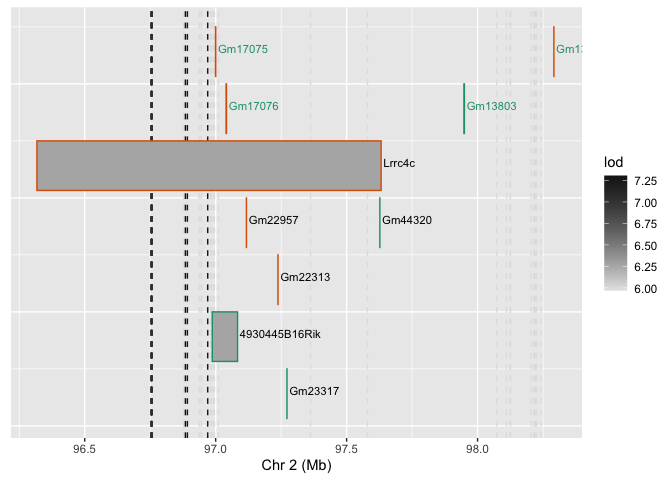The routine `gene_exon` examines individual genes and their exons. This example does not have exons, so there is just the figure of the gene. Exons would appear on different rows. Again, the vertical dashed lines correspond to SNPs with high LODs.

``````# Get Gene exon information.
out <- gene_exon(top_snps_tbl, gene_tbl)
summary(out, gene = out\$gene)``````
``````##            gene source type    start     stop strand
## 1 4930445B16Rik    MGI gene 96.98702 97.08356      -``````
``ggplot2::autoplot(out, top_snps_tbl)``